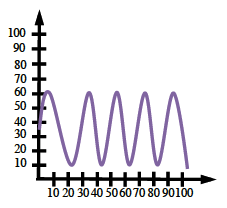### Home > PC > Chapter 5 > Lesson 5.2.2 > Problem5-65

5-65.Jade is having a wonderful time riding a merry-go-round. Her distance $d$ (in feet) from the fence nearest the entrance is given by the equation $d = 25 \operatorname { sin } ( \frac { \pi t } { 15 } ) + 35$ where $t$ is the time in seconds since the merry-go-round started to rotate.

1. How close can Jade be to the fence? How far?

What are the minimum and maximum values of the function?

2. How long does it take for the merry-go-round to complete one revolution?

This is the time for one cycle, or the period. You are given the frequency.
$\left(\text{frequency}\right)\left(\text{period}\right) = 2π$

3. How long before Jade is $60$ feet from the fence for the second time?

$f\left(t\right) = 60$
Find $t$.

4. Sketch the graph of $d$ vs. $t$. Label and scale (put numbers on) the axes appropriately.